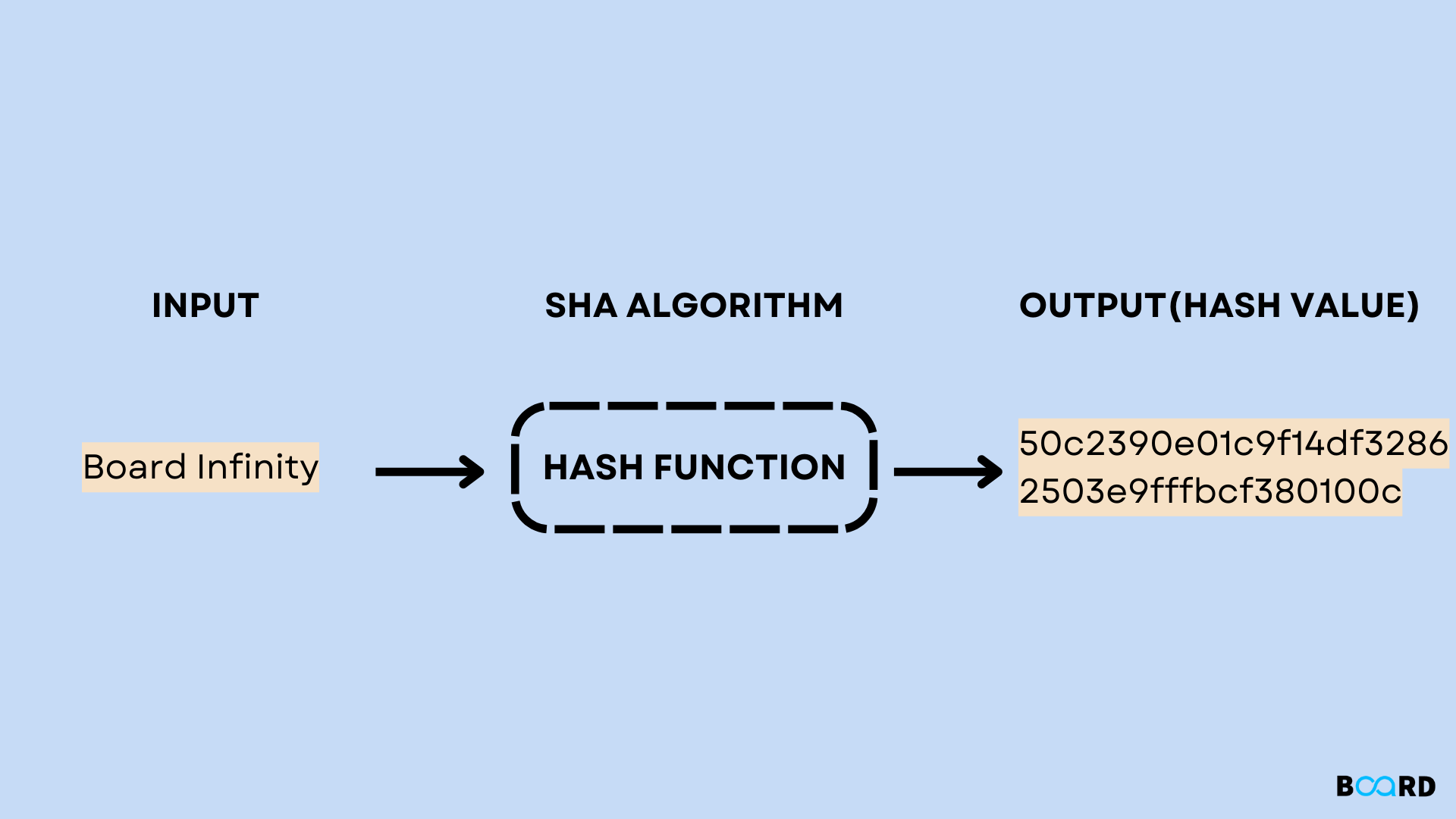# SHA-1 Hash Algorithm## Introduction

SHA-1 or Secure Hash Algorithm is a cryptographic hash function that takes a string as an input and generates a hash value of 160bit or 20 bytes. The hash value is generally called a message digest. This message digest is generally altered into a 40-decimal long hexadecimal value. Many tech giants like Google, Microsoft, and Apple is now considered insecure by US Federal Agencies since 2005. For calculating the hash value i.e, Message Digest java uses a MessageDigestClass which is present under the package of java.util.package. These algorithms are initialized in a static method called getInstance(). After selecting the algorithm the message digest value is calculated and the results are returned as a byte array.

BigInteger class is used, to convert the resultant byte array into its signum representation. This representation is then converted into a hexadecimal format to get the expected MessageDigest.

## Implementation

Below is the implementation of SHA -1 in java

 import java.math.BigInteger;import java.security.MessageDigest;import java.security.NoSuchAlgorithmException;// Main classpublic class Solution {    public static String encryptThisString(String input)    {           // handling exceptions        try {            // getInstance() method is called with algorithm SHA-1            MessageDigest md = MessageDigest.getInstance("SHA-1");             // digest() method is called to calculate message digest of the input string            byte[] messageDigest = md.digest(input.getBytes());             // Convert byte array into signum representation            BigInteger no = new BigInteger(1, messageDigest);             // Convert message digest into hexadecimal value            String hashtext = no.toString(16);             // Add preceding 0s to make it a 32 bit value            while (hashtext.length() < 32) {                hashtext = "0" + hashtext;            }            // return the HashText created            return hashtext;        }        // For specifying wrong message digest algorithms        catch (NoSuchAlgorithmException e) {            throw new RuntimeException(e);        }    }     // Driver code    public static void main(String args[]) throws NoSuchAlgorithmException{        System.out.print("HashCode Generated by SHA-1 for: ");         String str1 = "Board Infinity";        System.out.print("\n" + str1 + " : " + encryptThisString(str1));         String str2 = "Website";        System.out.println("\n" + str2 + " : " + encryptThisString(str2));    }}

The output obtained is as follows:

 HashCode Generated by SHA-1 for:Board Infinity : 50c2390e01c9f14df32862503e9fffbc380100cWebsite : 2e8a57cc5c472f4ac3b071979a38e80db7e59e87
write your code here: Coding Playground

## Conclusion

SHA-1 or Secure Hash Algorithm which generates the crtpographic hash value for the given input. This hash value is called message digest and it is 20 bytes long.